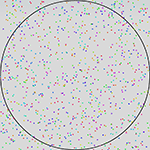MSTE
Office for Mathematics, Science, and Technology Education
College of Education @ University of Illinois

# MSTE Online Resource Catalog

## Estimation of PiShoot random points at a circle to approximate the value of Pi. Go to this resource Author: Evan Ramos Date Created: 7/27/1998 Last Modified: 6/16/2014
Detailed Description:

This applet is designed to approximate the value of pi. It accomplishes this purpose by firing random data points at a circle inscribed within a square. The probability of a data point landing within the circle is a ratio of the circle's area to the area of the square.

Where r equals the length of the radius of the circle, the area of the circle is then chr(960)*r*r. The diameter of the circle is the same as the length of each side of the square. Since the diameter of the circle, twice the radius, equals (2*r), the area of the square is equal to (2*r)*(2*r).

The probability of a point landing within the circle equals (chr(960)*r*r)/(2*r*2*r). By then performing algebra and removing (r*r) from the numerator and the denominator the simplified equation becomes P(landing in circle) = chr(960)/4.

Next, multiply both sides by 4.

Pi is now equivalent to four times the probability of a data point landing in the circle.

Resource Type:
Interactive (Lessons that include an interactive component, generally Java, JavaScript, Flash, or a TI program.)
Category:
Related Resources:
None specified yet
Other Information:
Original Java Applet by Nick Exner (7/27/1998)
JavaScript+HTML5 Remake by Evan Ramos (2014)
< Back